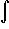#Interactive Real Analysis

Next | Previous | Glossary | Map

## 7.4. Lebesgue Integral

### Theorem 7.4.8: Riemann implies Lebesgue Integrable

If f is a bounded function defined on [a, b] such that f is Riemann integrable, then f is Lebesgue integrable andf(x) dx =[a,b] f(x) dx
The proof is simple. Recall that for a given function f we defined I*(f) to be the infimum over all upper sums and I*(f) to be the supremum over all lower sums.

Since every step function is also a simple function, every upper sum is a simple function that is bigger than f, and every lower function is a simple function less than f. Therefore:

I*(f)I*(f)LI*(f)LI*(f)
But if f is Riemann integrable, the first and last quantities agree, so that f must be Lebesgue integrable as well with the same value for the integral.
Next | Previous | Glossary | Map